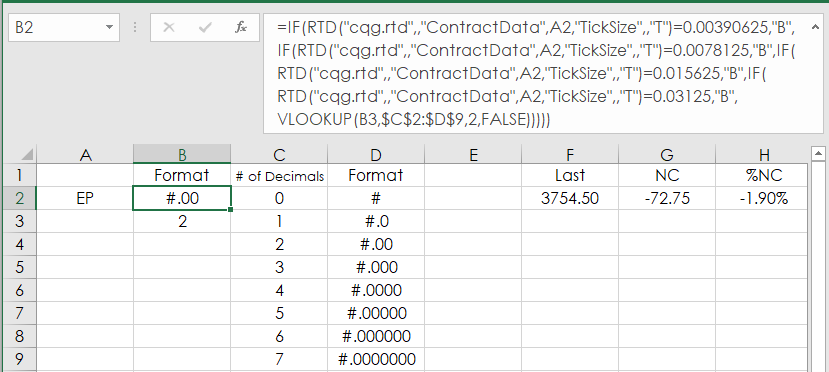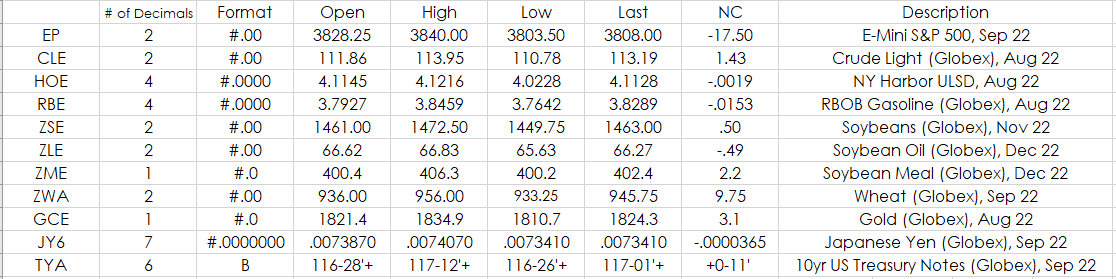# Excel Functions for Automatically Formatting Market Prices

When using RTD functions in Excel to pull in market prices you have to manually format the prices. Excel does not know that the E-mini S&P 500 price has two decimals places (i.e. 3909.25) and the Euro FX has 5 decimals places (i.e. 1.06525). Then, there is also formatting price issues with Fixed Income futures: Do we want the price in 32nds or decimals? And, if you do not manually set the correct number of decimals then the prices will jump back and forth sideways if the last price has zeroes at the end (i.e. EP displays 3909 and then 3909.25).

Ideally, the goal is you enter in the symbol (always use all capital letters) in Excel and Excel always formats the price with the correct number of decimals. This post walks you through setting up Excel to always displays prices with the correct number of decimals.

The first step is we need to know the tick size of the symbol:

`=RTD("cqg.rtd",,"ContractData","EP","TickSize",,"T")`

Returns 0.25

For Euro FX:

`=RTD("cqg.rtd",,"ContractData","EU6","TickSize",,"T")`

Returns 0.00005

For TYA:

`=RTD("cqg.rtd",,"ContractData","TYA","TickSize",,"T")`

Returns 0.015625

We can use the Excel Len() function to count the number of characters in the returned value for the tick size. Then, subtract 2 for the zero to the left of the decimal point and the decimal point and now we have the number of decimal places for the tick size.

For the E-mini S&P contract:

`=LEN(RTD("cqg.rtd",,"ContractData","EP","TickSize",,"T"))-2`

Returns 2

For the Natural Gas contract:

`=LEN(RTD("cqg.rtd",,"ContractData","NGE","TickSize",,"T"))-2`

Returns 3

Next, we will use the Excel Text function to format the price using the correct number of decimals.

The Text function only has one parameter for the formatting.

Formatting the E-mini S\$P contract you would use "#.00" to set the price to always be displayed using two decimals:

`=TEXT(RTD("cqg.rtd",,"ContractData","EP","LastTrade",,"T"),"#.00")`

At this point, we have a way to determine the number decimals and how to format the prices. Next, we use a table of possible parameters for formatting the price.

 0 # 1 #.0 2 #.00 3 #.000 4 #.0000 5 #.00000 6 #.000000 7 #.0000000

The table uses up to seven decimal places. The Japanese Yes contract (JY6) has seven decimal places.

Using the Excel VLOOKUP function we look up the number of decimals in the first column and return the parameter found in the second column to be used in the Text function.

For example, in the downloadable Excel sample cell B2 will display the Text parameter from the Lookup table for the symbol in cell A2:

`=TEXT(RTD("cqg.rtd",,"ContractData",A2,"LastTrade",,"T"),B2))`

There is one symbol, possibly more, that the price has no decimals and that symbol is YM for the E-mini Dow (Last price is 32150). To deal with this edge case cell B3 returns a 0 if there are no decimal places.

`=IF(LEN(RTD("cqg.rtd",,"ContractData",A2,"TickSize",,"T"))-2<0,0,LEN(RTD("cqg.rtd",,"ContractData",A2,"TickSize",,"T"))-2)`

Next, is dealing with Fixed Income prices.

This is a list of the tick sizes for CBOT Fixed Income products traded on Globex:

• TUA: 0.00390625
• FVA: 0.0078125
• TYA: 0.015625
• USA: 0.03125

Now, RTD Market Data labels (ContractData) have two parameters for prices. “T” returns a decimal and “B” returns a fraction:

`=RTD("cqg.rtd",,"ContractData","TYA","LastTrade",,"T") returned 116.671875` `=RTD("cqg.rtd",,"ContractData","TYA","LastTrade",,"B") returned 116-21'+`

Using the tick size for the Fixed Income products we can test for a Fixed Income product and return “B” if the symbol in cell A2 is a Fixed Income product.

`=IF(RTD("cqg.rtd",,"ContractData",A2,"TickSize",,"T")=0.00390625,"B",IF(RTD("cqg.rtd",,"ContractData",A2,"TickSize",,"T")=0.0078125,"B",IF(RTD("cqg.rtd",,"ContractData",A2,"TickSize",,"T")=0.015625,"B",IF(RTD("cqg.rtd",,"ContractData",A2,"TickSize",,"T")=0.03125,"B",))))`

Combining all of the above information the RTD formula below checks for a Fixed Income product first and if not then the number of decimals is determined in cell B3 and is looked up in the table \$C\$2:\$D\$9 for the Text formatting parameter.

`=IF(RTD("cqg.rtd",,"ContractData",A2,"TickSize",,"T")=0.00390625,"B",IF(RTD("cqg.rtd",,"ContractData",A2,"TickSize",,"T")=0.0078125,"B",IF(RTD("cqg.rtd",,"ContractData",A2,"TickSize",,"T")=0.015625,"B",IF(RTD("cqg.rtd",,"ContractData",A2,"TickSize",,"T")=0.03125,"B",VLOOKUP(B3,\$C\$2:\$D\$9,2,FALSE)))))`The downloadable Excel sample has all of the necessary RTD and Excel Functions including a sample portfolio.A tip: Hide the "# of Decimals" and "Format" columns and the Text format table for better screen real estate management.

Requires CQG Integrated Client or CQG QTrader, and Excel 2010 or higher. Excel has to be installed on the local computer, not in the cloud.